# Arduino Color Mixing Lamp using RGB LED and LDR

Published  May 22, 2018   1
R
Author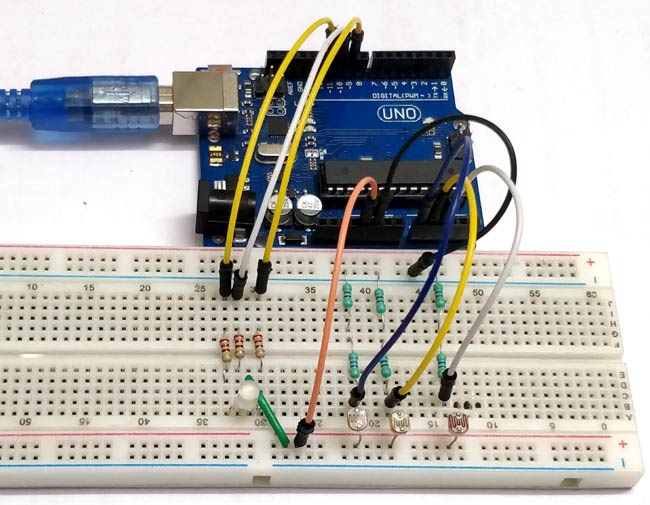What if we can generate different colors using a single RGB led and make our room’s corner more attractive? So, here is a simple Arduino based color mixing lamp which can change color when there is change in light in the room. So this lamp will automatically will changes its color according to the light conditions in the room.

Every color is the combination of Red, Green and Blue color. So we can generate any color by using red, green and blue colors .So, here we will vary PWM i.e. intensity of light on LDRs. That will further changes the intensity of red, green and blue color in RGB LED, and different colors will be produced.

Below table shows the color combinations with respective change in duty cycles.

### Materials required:

• 1 x Arduino UNO
• 3 x 220-ohm resistors
• 3 x 1-kilohm resistors
• Jumper wires
• 3 x LDRs
• 3 x colored strips (red, green, blue)
• 1 x RGB LED

### LDR:

We will use photoresistor (or light-dependent resistorLDR, or photo-conductive cell) here in this circuit. LDRs are made from semiconductor materials to enable them to have their light-sensitive properties. These LDRs or PHOTO RESISTORS works on the principle of “Photo Conductivity”. Now what this principle says is, whenever light falls on the surface of the LDR (in this case) the conductance of the element increases or in other words, the resistance of the LDR falls when the light falls on the surface of the LDR. This property of the decrease in resistance for the LDR is achieved because it is a property of semiconductor material used on the surface.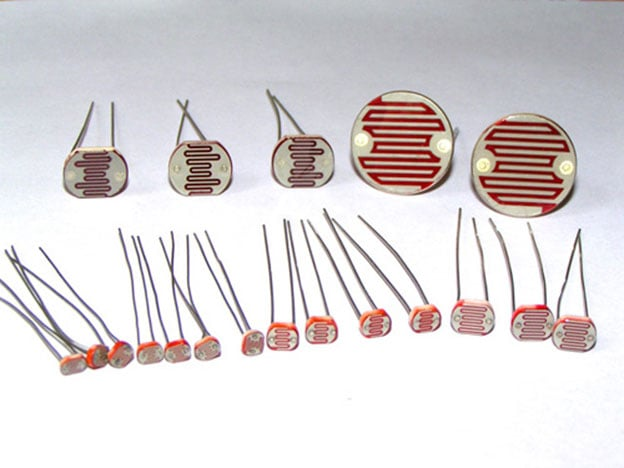Here three LDR sensors are used to control the brightness of individual Red, Green and Blue LED inside RGB Led. Learn more about controlling LDR with Arduino here.

### RGB LED:

There are two types of RGB LEDs, one is common cathode type (common negative) and other is common anode type (common positive) type. In CC (Common Cathode or Common Negative), there will be three positive terminals each terminal representing a color and one negative terminal representing all three colors.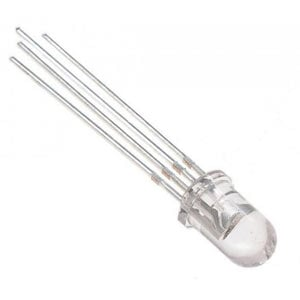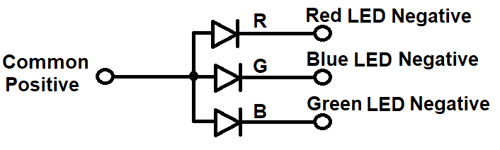In our circuit we are going to use CA (Common Anode or Common Positive) type. In Common Anode type, if we want RED LED to be ON in, we need to ground the RED LED pin and power the common positive. The same goes for all the LEDs. Learn here to interface RGB LED with Arduino.

### Circuit Diagram: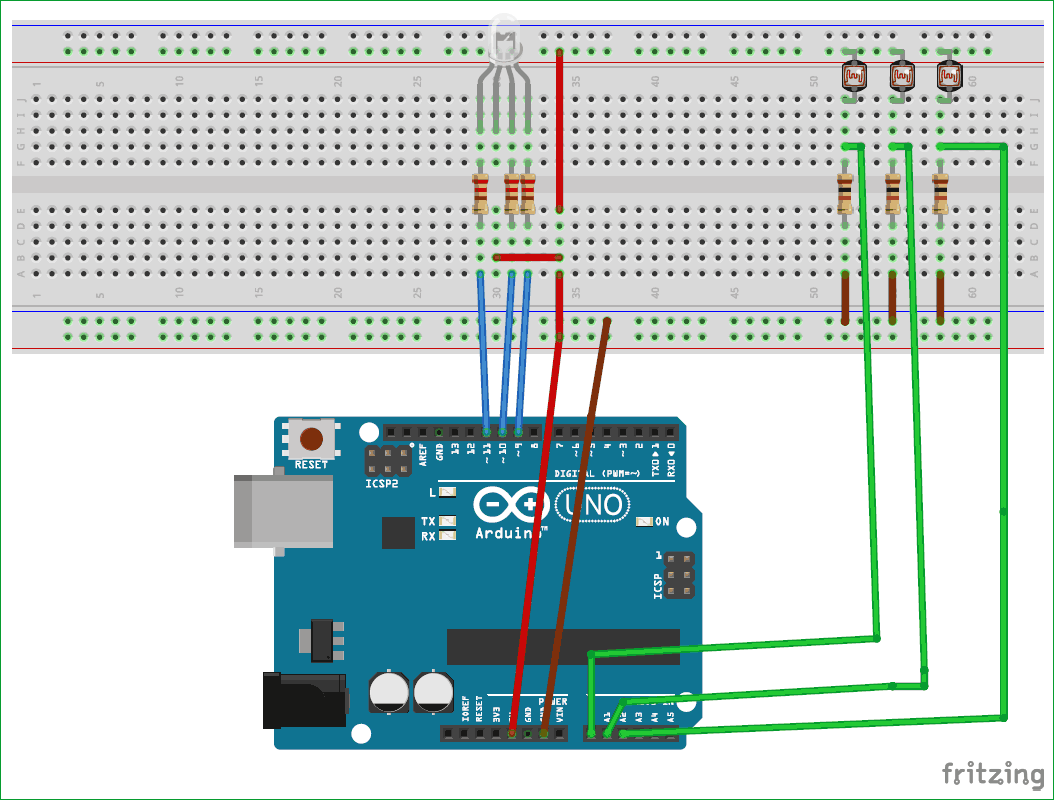The complete circuit diagram of this project is given above. The +5V and ground connection shown in the circuit diagram can be obtained from the 5V and ground pin of the Arduino. The Arduino itself can be powered from your laptop or through the DC jack using a 12V adapter or 9V battery.

We will use PWM to change brightness of RGB led. You can learn more about PWM here. Here are some PWM examples with Arduino:

### Programming Explanation:

First, we declare all the inputs and output pins as shown below.

```const byte red_sensor_pin = A0;
const byte green_sensor_pin = A1;
const byte blue_sensor_pin = A2;
const byte green_led_pin = 9;
const byte blue_led_pin = 10;
const byte red_led_pin = 11;```

Declare initial values of sensors and leds as 0.

```unsigned int red_led_value      = 0;
unsigned int blue_led_value     = 0;
unsigned int green_led_value    = 0;
unsigned int red_sensor_value   = 0;
unsigned int blue_sensor_value  = 0;
unsigned int green_sensor_value = 0;

void setup() {
pinMode(red_led_pin,OUTPUT);
pinMode(blue_led_pin,OUTPUT);
pinMode(green_led_pin,OUTPUT);
Serial.begin(9600);
}```

In loop section, we will take output of three sensors with analogRead(); function and store in three different variables.

```void loop() {
delay(50);
delay(50);

Print those values onto the serial monitor for debugging purpose

```    Serial.println("Raw Sensor Values:");
Serial.print("\t Red: ");
Serial.print(red_sensor_value);
Serial.print("\t Blue: ");
Serial.print(blue_sensor_value);
Serial.print("\t Green: ");
Serial.println(green_sensor_value);```

We will get 0-1023 values from the sensors but our Arduino PWM pins have 0-255 values as output. So we have to convert our raw values to 0-255. For that we have to divide raw values by 4 OR simply we can use mapping function of Arduino to convert these values.

```   red_led_value   = red_sensor_value / 4;   // define Red LED
blue_led_value  = blue_sensor_value / 4;  // define Blue LED
green_led_value = green_sensor_value / 4; // define Green Led```

Print mapped values to serial monitor

```    Serial.println("Mapped Sensor Values:");
Serial.print("\t Red: ");
Serial.print(red_led_value);
Serial.print("\t Blue: ");
Serial.print(blue_led_value);
Serial.print("\t Green: ");
Serial.println(green_led_value);```

Use analogWrite() to set output for RGB LED

```  analogWrite(red_led_pin,red_led_value);   // indicate red LED
analogWrite(blue_led_pin,blue_led_value);  // indicate blue LED
analogWrite(green_led_pin,green_led_value); // indicate green```

### Working of Arduino Color Mixing Lamp: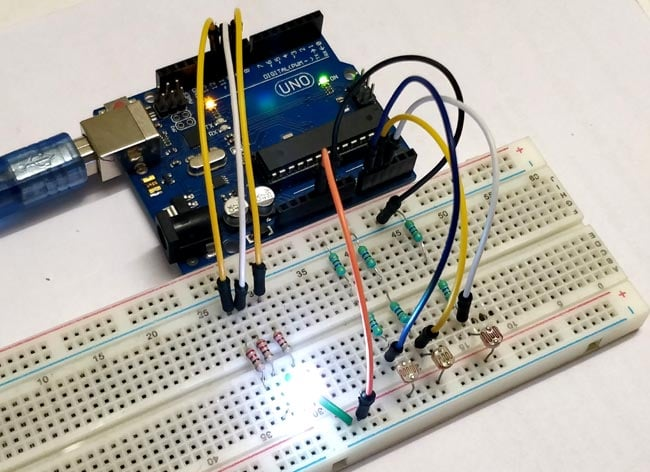As we are using three LDR’s so, when light incident on these sensors ,it’s resistance changes as a result voltages also changes at analog pins of Arduino which is acting as a input pins for sensors.

When intensity of light changes on these sensors, it’s respective led in RGB will glow with amount of resistance changing and we have different color mixing in RGB led using PWM.

Code

const byte red_sensor_pin = A0;
const byte green_sensor_pin = A1;
const byte blue_sensor_pin = A2;
const byte green_led_pin = 9;
const byte blue_led_pin = 10;
const byte red_led_pin = 11;

unsigned int red_led_value      = 0;
unsigned int blue_led_value     = 0;
unsigned int green_led_value    = 0;
unsigned int red_sensor_value   = 0;
unsigned int blue_sensor_value  = 0;
unsigned int green_sensor_value = 0;

void setup() {
pinMode(red_led_pin,OUTPUT);
pinMode(blue_led_pin,OUTPUT);
pinMode(green_led_pin,OUTPUT);
Serial.begin(9600);
}

void loop() {
delay(50);
delay(50);
// print those values onto the serial monitor
Serial.println("Raw Sensor Values:");
Serial.print("\t Red: ");
Serial.print(red_sensor_value);
Serial.print("\t Blue: ");
Serial.print(blue_sensor_value);
Serial.print("\t Green: ");
Serial.println(green_sensor_value);
// convert from 0-1023 to 0-255
red_led_value   = red_sensor_value / 4;   // define Red LED
blue_led_value  = blue_sensor_value / 4;  // define Blue LED
green_led_value = green_sensor_value / 4; // define Green LEd
// print mapped values to serial monitor
Serial.println("Mapped Sensor Values:");
Serial.print("\t Red: ");
Serial.print(red_led_value);
Serial.print("\t Blue: ");
Serial.print(blue_led_value);
Serial.print("\t Green: ");
Serial.println(green_led_value);
// use analogWrite() to set output for RGB LED
analogWrite(red_led_pin,red_led_value);   // indicate red LED
analogWrite(blue_led_pin,blue_led_value);  // indicate blue LED
analogWrite(green_led_pin,green_led_value); // indicate green
}

Video

Tags